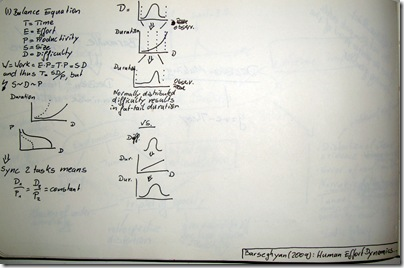## Human Effort Dynamics and Schedule Risk Analysis (Barseghyan, 2009)Barseghyan, Pavel: Human Effort Dynamics and Schedule Risk Analysis; in:  PM World Today, Vol. 11(2009), No. 3.
http://www.pmforum.org/library/papers/2009/PDFs/mar/Human-Effort-Dynamics-and-Schedule-Risk-Analysis.pdf

Barseghyan researched extensively the human dynamics within project work.  He has formulated a system of intricate mathematics quite similar to Boyle’s law and the other gas laws.  He establishes a simple set of formulas to schedule the work of software developers.

T = Time, E = Effort, P = Productivity, S = Size, and D = Difficulty
Then W = E * P = T * P = S * D, and thus T = S*D/P

But and now it gets tricky S~D~P are correlated!

The author has collected enough data to show the typical curves between Difficulty –> Duration and Difficulty –> Productivity.  To schedule and synchronise two tasks the D/P ratio has to be constant between these two tasks.

Barseghyan then continues to explore the details between Difficulty and Duration.  He argues that the common notion of bell-shaped distributions is flawed because of the non linear relationship between Difficulty –> Duration  [note that his curves have a segment of linearity followed by some exponential part.  If the Difficulty bell curve is transformed into the Diffculty–>Duration probability function using that non-linear transformation formula it looses is normality and results in a fat-tail distribution.  Therefore Barseghyan argues, the notion of using bell-shaped curves in planning is wrong.

### 3 Responses to “Human Effort Dynamics and Schedule Risk Analysis (Barseghyan, 2009)”

1.towel rails sagt: